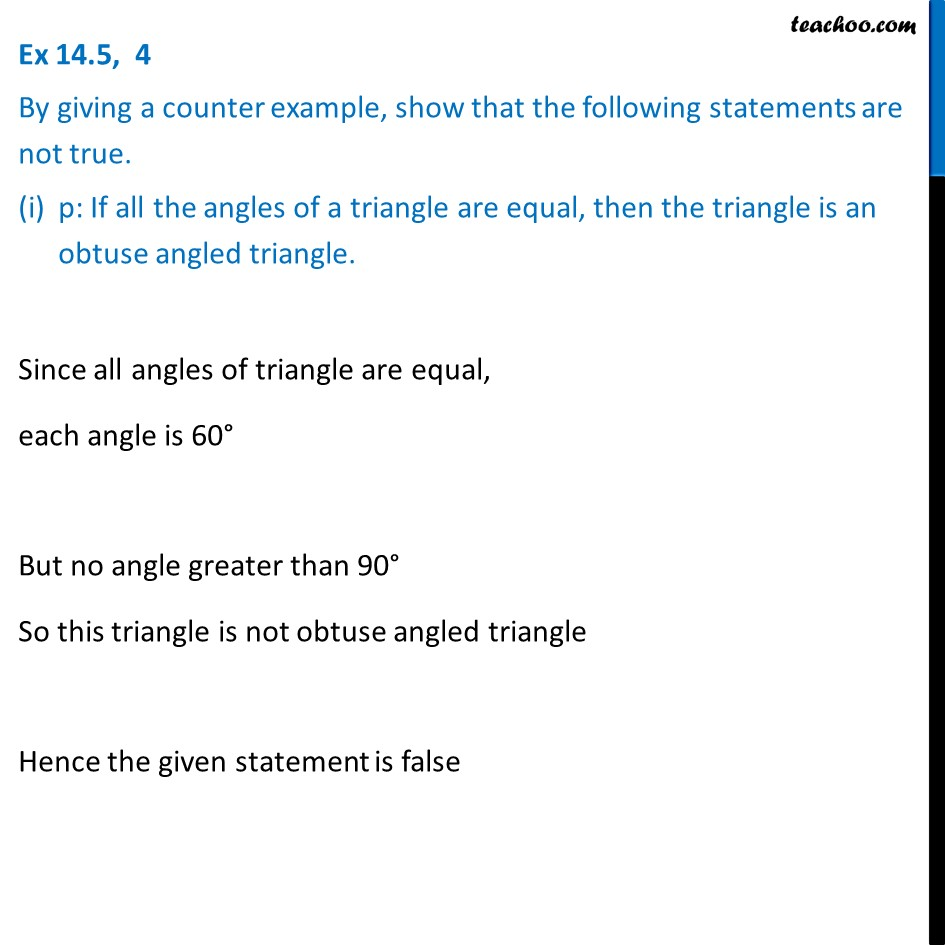Ex 14.5

Chapter 14 Class 11 Mathematical Reasoning (Deleted)
Serial order wise### Transcript

Ex 14.5, 4 By giving a counter example, show that the following statements are not true. p: If all the angles of a triangle are equal, then the triangle is an obtuse angled triangle. Since all angles of triangle are equal, each angle is 60° But no angle greater than 90° So this triangle is not obtuse angled triangle Hence the given statement is false